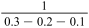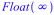Numeric Computation - How Does Maple Compare? - Maplesoft
Compare: Numeric Computation

In this video: Numeric Computation – How Does Maple Compare?

Maple provides high-performance, industry-standard routines for numeric computations.

• Maple includes platform-optimized industry-standard routines, including LAPACK and ATLAS for linear algebra, Numerical Algorithms Group (NAG) routines for linear algebra, equation solving, optimization, and more, and GMP for fast arbitrary precision arithmetic. These solvers are seamlessly integrated into Maple's high-level solvers and are called automatically when needed.
• Maple supports both hardware (double) precision and infinite precision computations. By increasing the precision, round-off error is reduced and problems of catastrophic cancellation can be avoided.
• Maple's default numeric environment propagates less error than ordinary IEEE/754 implementations. For example, in Maple, the expressionis evaluated to, which is treated correctly by subsequent calculations. Other systems produce an extremely large but finite number, which is then erroneously incorporated into further calculations.
• Maple extends the abilities and speed of its numeric solvers by applying a hybrid symbolic-numeric approach.

• If a problem is in a form that cannot be solved by standard numerical or symbolic approaches, Maple attempts to transform the problem symbolically into an equivalent form, which is amenable to numerical methods.
• Hybrid techniques are employed to select appropriate starting values for numerical solvers, allowing them to arrive at an answer more quickly.
• Hybrid approaches are fully integrated into the numeric solver algorithms and are applied automatically as needed.
• Maple includes the ability to externally compile custom numeric routines for more efficient computations. This functionality is built in; no additional toolboxes are required. The compiler supports an autocompile option for procedures that causes Maple to automatically compile the procedure on first execution as well as explicit compilation.
• Maple lets you control the number of digits displayed in the result, independent of the number used in the calculation. You can display numeric results using a variety of standard formats.